Next: Application of function Pearson7 Up: Method of determination of Previous: Equation and Scherrer constant

# Method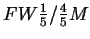of determination of Grain Size Distribution from a Diffraction Line Profile

Quantity of FWHM(Full Width at Half Maximum) is a commonly used crystallographic parameter, mainly due to clear definition and ease of determination. In case of crystalline powders containing grains of same size (mono-dispersive), there exists a simple relation between FWHMand the grain size, called Scherrer equation. In a more realistic case of polidisperse powders, single parameter FWHM is not sufficient to determine both properties of Grain Size Distribution: it's average <R> and dispersion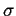. In order to derive two unknowns, two equations are needed - that's why determination of full Grain Size Distribution requires two line widths to be used: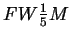and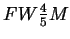- measured at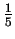and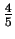of line maximum, respectively.

Detailed derivation ofmethod is skipped here and can be found in .

We assume gamma Grain Size Distribution of crystallites: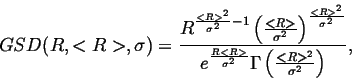(2)

We present expressions that can be readily used in order to obtain average size1 of the grain in a powder and dispersion of the GSD as a function of measured values ofand:

 =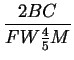(3)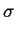=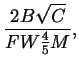where auxiliary variables A,B and C are: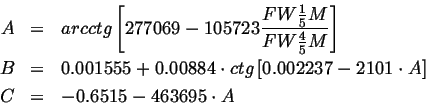Figure 2 shows examples of Grain Size Distribution determinations performed for ab initio calculated diffraction patterns. Theoretical diffraction patterns were obtained with log-normal Grain Size Distribution assumed. After background subtraction, widths of (111) and (113) lines have been measured atandtheir maximum. Then expressions 3 have been used in order to obtain GSD parameters <R> i. Having parameters <R> iwe have drawn Grain Size Distribution (2) obtained seen on right-hand side of the Fig. 2 (red curve) together with original GSD used during ab initio calculations of the diffraction pattern (black curve). Fig. 2 shows that gamma distribution of sizes used in derivation of themethod well reflects shape of log-norm distribution, however differs from the latter in mathematical properties, and is certainly sufficient for evaluations of experimental data. One has to state, however, that every quantitative analysis of the diffraction profile, includingmethod, is sensitive to any deformation of line, e.g. resulting from stacking faults or lattice strains.

Figure: Examples of application of method. Powder diffraction patterns of SiC (Fig. I,II,III a) have been calculated ab initio for powder with log-normal Grain Size Distribution (black curve on Fig. I,II,III b). After background subtraction, widths of lines (I:(111), II:(111), III:(113)) atandof their maxima have been measured. Expressions (3) have been used to determine the GSD (red curves on Fig. I,II,III b) from the measured widths. Assumed inmethod gamma distribution of crystallite sizes fairly reflects shape of log-norm distribution used for patterns calculation.
 I a)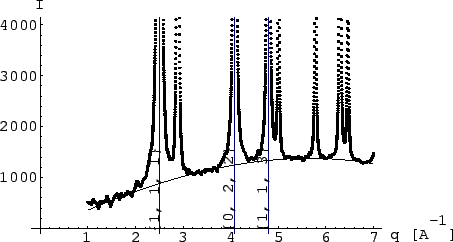I b)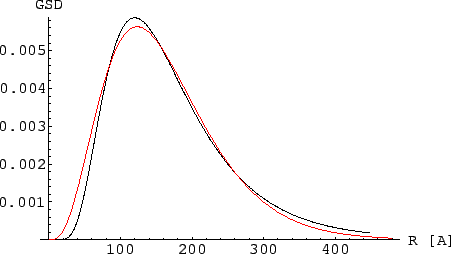II a)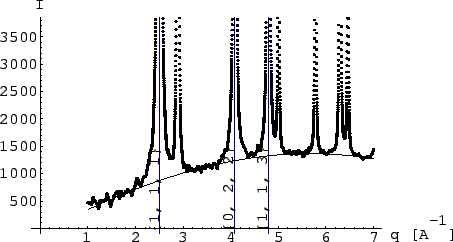II b)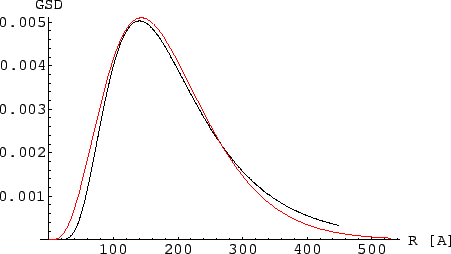III a)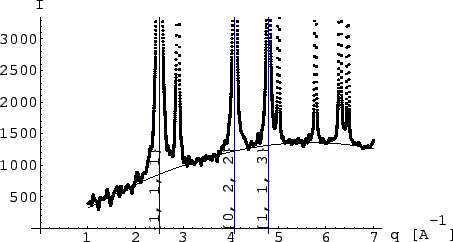III b)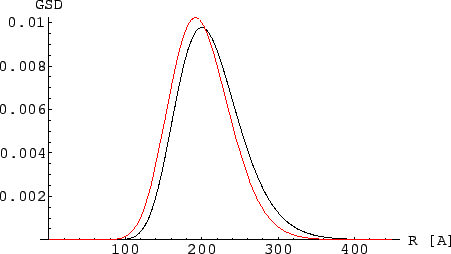Another example of usage of theis given on Fig.3. It shows standard GSD evaluation for BN nanocrystals from profile of (111), (220) and (311) diffraction lines. Standard deviations do not exceed 1% for average sizes determined and 4% for dispersions. Such a small deviations are due to good quality of diffraction data (smooth experimental curves).

Figure: Examples of application of methodto the experimantal data. (a) Powder diffraction pattern of nanocrystalline BN have been evaluated (peak 220 shown). (b) Resulting Grain Size Distribution functions show anisotropy of average shape of nanocrystals evaluated. Obtained values are: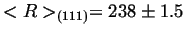Å,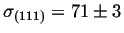Å,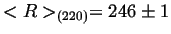Å,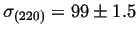Å,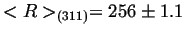Å,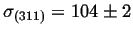Å. (a) Theoretical peak profiles calculated for obtained GSD (solid line) fits tightly corresponding experimental data (dots).
 a)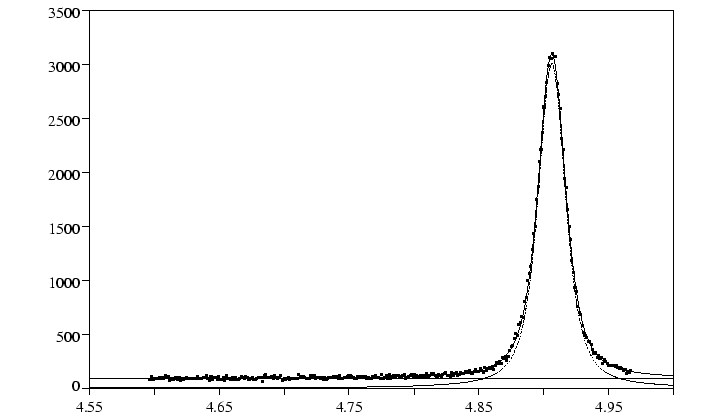b)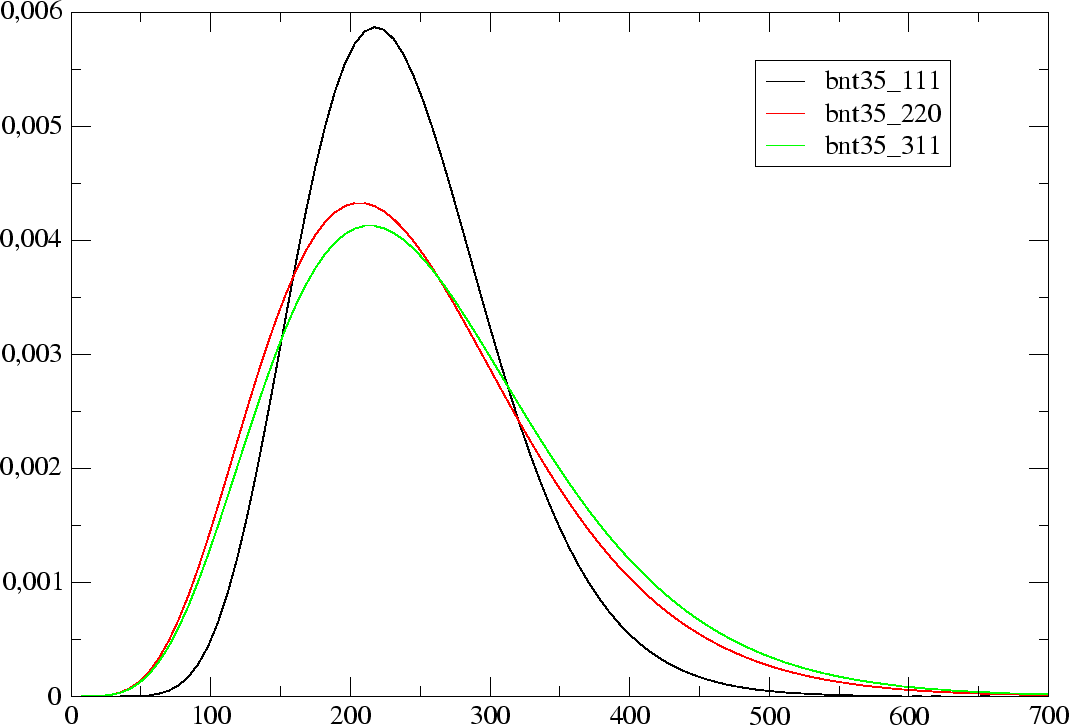In next section a practical supplement to themethod is presented. It allows for direct application of parameters obtained during fitting Pearson7 curve to the experimental peak profile. Curve Pearson7 is commonly used in popular crystallographic software.

SubsectionsNext: Application of function Pearson7 Up: Method of determination of Previous: Equation and Scherrer constant
roman pielaszek 2003-03-17